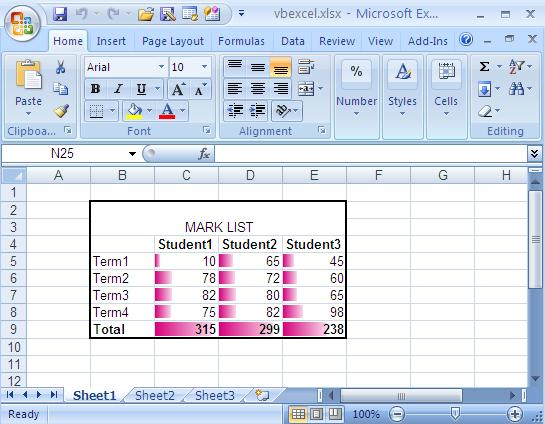VB.Net Tutorial

# Excel DataBar in VB.NET

The program presented below demonstrates how to perform commonly used formatting operations in an Excel spreadsheet. The main objective of this program is to format a page in Excel, particularly by entering data for a Mark List and applying various formatting techniques.

To begin, the program utilizes the MERGE function to merge cells and create a prominent heading for the Mark List. By combining multiple cells into a single cell, the heading becomes visually distinct and effectively conveys the purpose of the document. Additionally, specific formatting attributes such as bold font style are applied to highlight the students' names and total marks, further emphasizing their importance in the Mark List. Furthermore, a border is applied to the entire mark list section, enhancing its visual structure and making it more visually appealing and organized.

After you run the source code you will get an Excel file its look like in the following picture.As a final touch, the program selects a designated range within the Excel spreadsheet and adds a DataBar formatting feature. The DataBar serves as a visual representation of the data within the selected range, allowing for easy identification and comparison of values. The choice of color for the DataBar can be customized based on preference and intended visual impact.

Upon executing the provided source code, an Excel file will be generated, closely resembling the appearance depicted in the accompanying picture. This output reflects the successful implementation of the formatting operations described above, resulting in a well-structured and visually appealing Mark List. The applied formatting techniques enhance the clarity and readability of the data, facilitating efficient analysis and interpretation.

Full Source VB.NET
Imports Excel = Microsoft.Office.Interop.Excel Public Class Form1 Private Sub Button1_Click(ByVal sender As System.Object, _ ByVal e As System.EventArgs) Handles Button1.Click Dim xlApp As Excel.Application Dim xlWorkBook As Excel.Workbook Dim xlWorkSheet As Excel.Worksheet Dim misValue As Object = System.Reflection.Missing.Value Dim chartRange As Excel.Range xlApp = New Excel.ApplicationClass xlWorkBook = xlApp.Workbooks.Add(misValue) xlWorkSheet = xlWorkBook.Sheets("sheet1") 'add data xlWorkSheet.Cells(4, 2) = "" xlWorkSheet.Cells(4, 3) = "Student1" xlWorkSheet.Cells(4, 4) = "Student2" xlWorkSheet.Cells(4, 5) = "Student3" xlWorkSheet.Cells(5, 2) = "Term1" xlWorkSheet.Cells(5, 3) = "10" xlWorkSheet.Cells(5, 4) = "65" xlWorkSheet.Cells(5, 5) = "45" xlWorkSheet.Cells(6, 2) = "Term2" xlWorkSheet.Cells(6, 3) = "78" xlWorkSheet.Cells(6, 4) = "72" xlWorkSheet.Cells(6, 5) = "60" xlWorkSheet.Cells(7, 2) = "Term3" xlWorkSheet.Cells(7, 3) = "82" xlWorkSheet.Cells(7, 4) = "80" xlWorkSheet.Cells(7, 5) = "65" xlWorkSheet.Cells(8, 2) = "Term4" xlWorkSheet.Cells(8, 3) = "75" xlWorkSheet.Cells(8, 4) = "82" xlWorkSheet.Cells(8, 5) = "98" xlWorkSheet.Cells(9, 2) = "Total" xlWorkSheet.Cells(9, 3) = "315" xlWorkSheet.Cells(9, 4) = "299" xlWorkSheet.Cells(9, 5) = "238" chartRange = xlWorkSheet.Range("b2", "e3") chartRange.Merge() chartRange.FormulaR1C1 = "MARK LIST" chartRange.HorizontalAlignment = 3 chartRange.VerticalAlignment = 3 chartRange = xlWorkSheet.Range("b4", "e4") chartRange.Font.Bold = True chartRange = xlWorkSheet.Range("b9", "e9") chartRange.Font.Bold = True chartRange = xlWorkSheet.Range("b2", "e9") chartRange.BorderAround(Excel.XlLineStyle.xlContinuous, _ Excel.XlBorderWeight.xlMedium, Excel.XlColorIndex. _ ColorIndexAutomatic,Excel.XlColorIndex.xlColorIndexAutomatic) chartRange = xlWorkSheet.Range("c5", "e9") chartRange.FormatConditions.AddDatabar() chartRange.FormatConditions(chartRange.FormatConditions.Count) _ .ShowValue = True chartRange.FormatConditions(chartRange.FormatConditions.Count). _ SetFirstPriority() chartRange.FormatConditions(1).BarColor.color = 8061142 chartRange.FormatConditions(1).BarColor.TintAndShade = 0 xlWorkSheet.SaveAs("C:\vbexcel.xlsx") xlWorkBook.Close() xlApp.Quit() releaseObject(xlApp) releaseObject(xlWorkBook) releaseObject(xlWorkSheet) MsgBox("File created !") End Sub Private Sub releaseObject(ByVal obj As Object) Try System.Runtime.InteropServices.Marshal.ReleaseComObject(obj) obj = Nothing Catch ex As Exception obj = Nothing Finally GC.Collect() End Try End Sub End Class

### Conclusion

By using the capabilities of the Excel object model and employing the appropriate formatting functions, we can achieve consistent and professional-looking Excel documents. The ability to seamlessly format data, merge cells, apply font styles, add borders, and utilize advanced features like DataBars empowers users to create visually compelling and meaningful representations of their data.